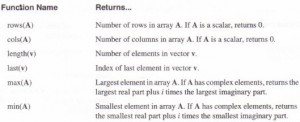# Vector and matrix functions MathCad Help

Mathcad includes functions for manipulating arrays in ways that are common in linear algebra. These functions are intended for use with vectors and matrices. If a function is not explicitly set up to take a vector or matrix argument, it is inappropriate to supply one to it as an argument. Note that functions which expect vectors always expect column vectors rather than row vectors. To change a row vector into a column vector, use the transpose operator [Ctrl]l.

The following tables list Mathcad’s vector and matrix functions. In these tables,

• A and B are arrays, either vector or matrix.
• v is a vector.
• M and N are square matrices.
• z is a scalar expression.
• Names beginning with m, n, i or j are integers.

Size and scope of an array

Mathcad provides several functions that return information about the size of an array and its elements. Figure 10-10 shows how these functions are used.Special types of matrices

You can use the following functions to derive from an array or scalar a special type or form of a matrix. Some of these functions are available only in Mathcad Professional.

Special characteristics of a matrix

You can use the functions in the following table to find the trace, rank, norms, and condition numbers of a matrix. Most of these functions are available only in Mathcad Professional.

Forming new matrices

Mathcad provides two functions for joining matrices together, either side by side, or one on top of the other. Mathcad also provides a function for filling in a matrix with values of a predefined function, and a function for extracting a smaller matrix from a larger one. Figure 10-12 and Figure 10-13 show some examples.

Eigenvalues and eigenvectors

Mathcad provides functions for working with eigenvalues and eigenvectors of a matrix. The eigenvecs function, available in Mathcad Professional, obtains all the eigenvectors at once. If you’re using Mathcad Professional, you’ll also have access to genvals and
genvecs for finding the generalized eigenvalues and eigenvectors. Figure 10-14 shows how some of these functions are used.

A matrix containing the normalized eigenvectors corresponding to the eigenvalues in v, the vector returned by genvais. The nth column of this matrix is the eigenvector x satisfying the generalized eigenvalue problem M . x = V n . N . x . Matrices M and N are real valued square matrices having the same number of columns.

Decomposition

Mathcad Professional offers some additional functions for performing the cholesky decomposition, the QR decomposition, the LU decomposition, and the singular value decomposition of a matrix. Some of these functions return two or three matrices joined together as one large matrix. Use submatrix to extract these two or three smaller matrices. Figure 10-16 shows an example.

Solving a linear system of equations

With Mathcad Professional, you’ll be able to use the lsolve function to solve a linear system of equations. Figure 10-17 shows an example. Note that the argument M for lsolve must be a matrix that is neither singular nor nearly singular. A matrix is singular if its determinant is equal to zero. A matrix is nearly singular if it has a high condition number. You may want to use one of the functions described in”Special charact,eristics of a matrix” on page 198 to find the condition number of a matrix.

Alternatively, you can solve a system of linear equations by using matrix inversion as shown in the lower right comer of Figure 10-9. For other numerical solving techniques in Mathcad, see Chapter 15, “Solving Equations.” For symbolic solutions of systems of equations, see Chapter 17, “Symbolic Calculation.”

Posted on November 20, 2015 in Vectors and Matrices